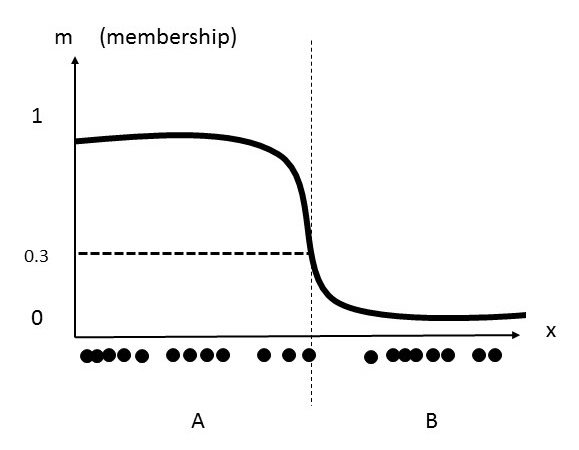# Fuzzy Clustering: Definition

Share on

Clustering > Fuzzy Clustering

## What is Fuzzy Clustering?

Fuzzy clustering is a clustering method where data points can belong in more than one group (“cluster”). Clustering divides data points into groups based in similarity between items and looks to find patterns or similarity between items in a set; Items in clusters should be as similar as possible to each other and as dissimilar as possible to items in other groups . Computationally, it’s much easier to create fuzzy boundaries than it is to settle on one cluster for one point.An example of fuzzy clustering, where the middle point can belong to either group A or B .

In “hard” clustering, each data point can only be in one cluster. In “soft” or “fuzzy” clustering, data points can belong to more than one group. Fuzzy clustering uses least-squares solutions to find the optimal location for any data point. This optimal location may be in a probability space between two (or more) clusters.

Fuzzy clustering is very similar to atomic orbitals and electron behavior: an electron isn’t in a single location but only has a probability of being in a particular orbital shell. If you think of orbital shells as “clusters” and electrons as “data points” (where each data point is assigned a probability for being located in a particular cluster), then you’ve got a basic grasp of the fundamentals of fuzzy clustering.

## Algorithms

Fuzzy clustering algorithms are divided into two areas: classical fuzzy clustering and shape-based fuzzy clustering.

### Classical fuzzy clustering algorithms.

1. Fuzzy C-Means algorithm (FCM). This widely-used algorithm is practically identical to the K-Means algorithm. A data point can theoretically belong to all groups, with a membership function (also called a membership grade) between 0 and 1, where: 0 is where the data point is at the farthest possible point from a cluster’s center and 1 is where the data point is closest to the center. Subtypes include Possibilistic C-Means (PCM), Fuzzy Possibilistic C-Means (FPCM) and Possibilistic Fuzzy C-Means (PFCM).
2. Gustafson-Kessel (GK) algorithm: associates a data point with a cluster and a matrix. While C-means assumes the clusters are spherical, GK has elliptical-shaped clusters.
3. Gath-Geva algorithm (also called Gaussian Mixture Decomposition): similar to FCM, but clusters can have any shape.

### Shape-based fuzzy clustering algorithms.

1. Circular shaped: circular-shaped (CS) algorithms are what constrains data point to a circular shape. When this algorithm is incorporated into Fuzzy C-Means it’s called CS-FCM.
2. Elliptical shaped: an algorithm that constrains points to elliptical shapes. Used in the GK algorithm.
3. Generic shaped: most real life objects are neither circular not elliptical; the generic alorithm allows for clusters of any shape.

## References:

 Suganya, R. & Shanthi, R. Fuzzy C-Means Algorithm — A Review. International Journal of Scientific and Research Publications, Volume 2, Issue 11, November 2012 1
 By Danielcschossow – Own work, CC BY-SA 4.0, https://commons.wikimedia.org/w/index.php?curid=58428026

CITE THIS AS:
Stephanie Glen. "Fuzzy Clustering: Definition" From StatisticsHowTo.com: Elementary Statistics for the rest of us! https://www.statisticshowto.com/fuzzy-clustering/
---------------------------------------------------------------------------Need help with a homework or test question? With Chegg Study, you can get step-by-step solutions to your questions from an expert in the field. Your first 30 minutes with a Chegg tutor is free!